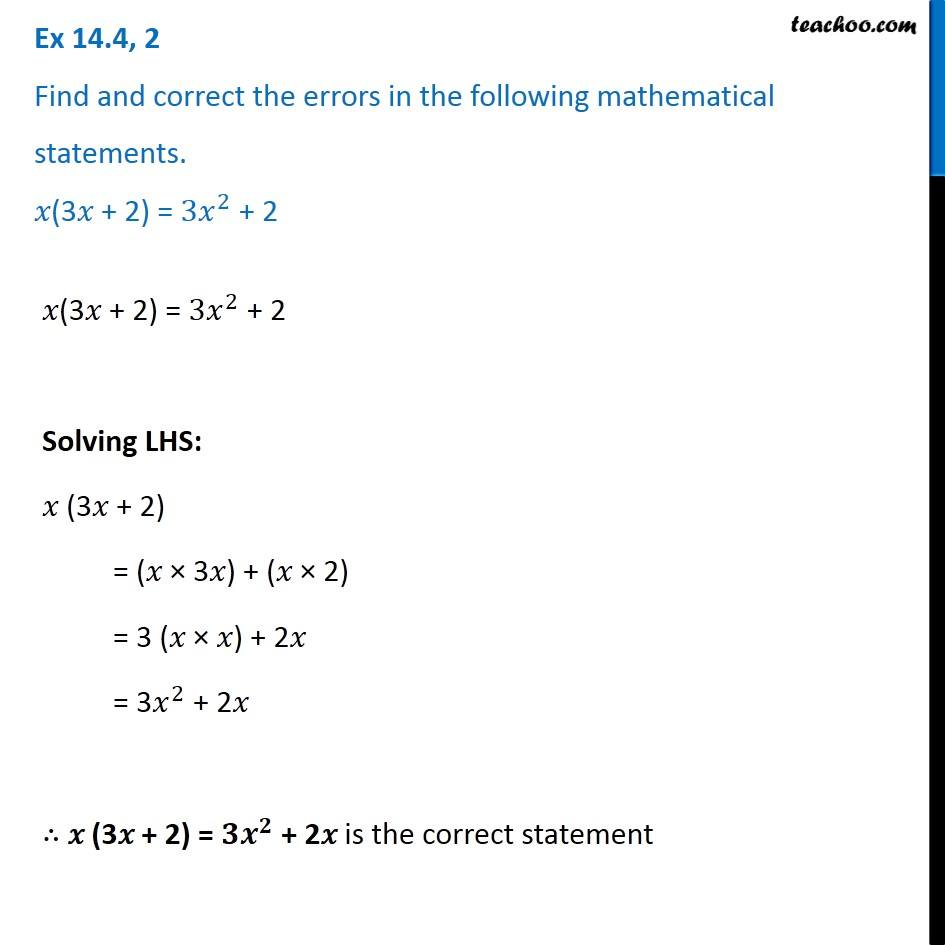Identify and correct errors in the sentences

Chapter 12 Class 8 Factorisation
Serial order wiseLearn in your speed, with individual attention - Teachoo Maths 1-on-1 Class

### Transcript

Question 2 Find and correct the errors in the following mathematical statements. 𝑥(3𝑥 + 2) = 〖3𝑥〗^2 + 2 𝑥(3𝑥 + 2) = 〖3𝑥〗^2 + 2 Solving LHS: 𝑥 (3𝑥 + 2) = (𝑥 × 3𝑥) + (𝑥 × 2) = 3 (𝑥 × 𝑥) + 2𝑥 = 3𝑥^2 + 2𝑥 ∴ 𝑥 (3𝑥 + 2) = 〖𝟑𝒙〗^𝟐 + 2𝑥 is the correct statement